Grade 10 questions on how to add and subtract expressions with radicals and their solutions are presented.

## Definition

Radical expressions are like if they have the same index and the same radicand.
Examplesare like radicals because they have the same index (root number which is 3) and the same radicand (number under the radical which is 5.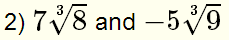are not like radicals because they have different radicands 8 and 9.are like radicals because they have the same index (2 for square root) and the same radicand 2 x.

Examples
Simplify the following expressions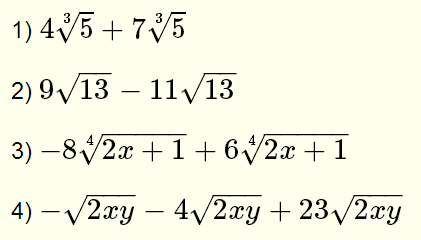Solutions to the Above Examples
The above expressions are simplified by first factoring out the like radicals and then adding/subtracting.## More Examples

Simplify the following expressionsSolutions to Above Examples

The above expressions are simplified by first transforming the unlike radicals to like radicals and then adding/subtracting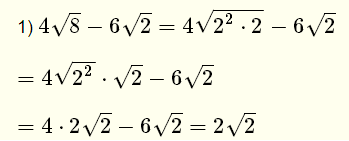When it is not obvious to obtain a common radicand from 2 different radicands, decompose them into prime numbers. Decompose 12 and 108 into prime factors as follows.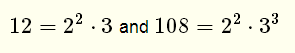We now substitute 12 and 108 by their prime factors and simplify## Questions With Solutions

Simplify the following expressions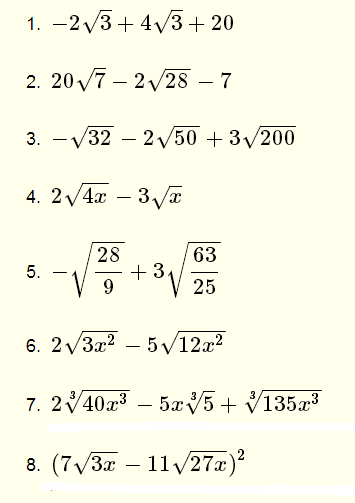## Solutions to the Above Questions3. The 3 radicands in the given expression -√ 32 - 2√ 50 + 3√ 200 are different but note that 32, 50 and 200 may be written as 2 times a number that is a perfect square as follows: 32=2 * 16, 50=2 * 25 and 100=2 * 100. Substitute in the given expression and simplify.Decompose 28 and 63 into prime factors as follows: 28=2 2 * 7 , 63=3 2 * 7 and substitute into the given expression and simplify7.More High School Math (Grades 10, 11 and 12) - Free Questions and Problems With Answers
More Middle School Math (Grades 6, 7, 8, 9) - Free Questions and Problems With Answers
More Primary Math (Grades 4 and 5) with Free Questions and Problems With Answers